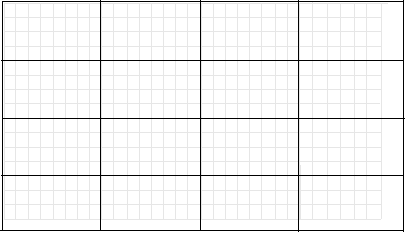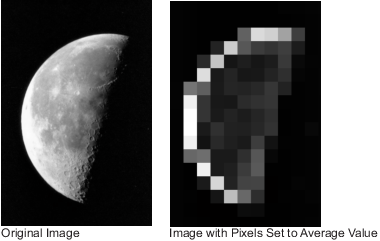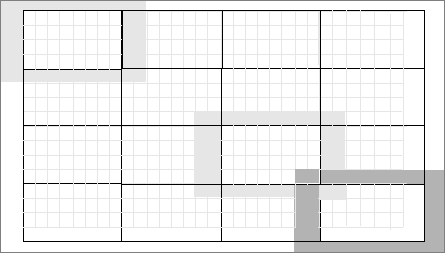## Distinct Block Processing

In distinct block processing, you divide a matrix into `m`-by-`n` sections. These sections, or distinct blocks, overlay the image matrix starting in the upper left corner, with no overlap. If the blocks do not fit exactly over the image, you can add padding to the image or work with partial blocks on the right or bottom edges of the image. The following figure shows a 15-by-30 matrix divided into 4-by-8 blocks. The right and bottom edges have partial blocks. You can process partial blocks as is, or you can pad the image so that the resulting size is 16-by-32. For more information, see Apply Padding. (To operate on an image a pixel at a time, rather than a block at a time, use the sliding neighborhood processing function. For more information, see Sliding Neighborhood Operations.)

Image Divided into Distinct Blocks### Implement Block Processing Using the blockproc Function

To perform distinct block operations, use the `blockproc` function. The `blockproc` function extracts each distinct block from an image and passes it to a function you specify for processing. The `blockproc` function assembles the returned blocks to create an output image.

For example, the commands below process image `I` in 25-by-25 blocks with the function `myfun`. In this case, the `myfun` function resizes the blocks to make a thumbnail. (For more information about function handles, see Create Function Handle. For more information about anonymous functions, see Anonymous Functions.)

```myfun = @(block_struct) imresize(block_struct.data,0.15); I = imread('tire.tif'); I2 = blockproc(I,[25 25],myfun);```

Note

Due to block edge effects, resizing an image using `blockproc` does not produce the same results as resizing the entire image at once.

The example below uses the `blockproc` function to set every pixel in each 32-by-32 block of an image to the average of the elements in that block. The anonymous function computes the mean of the block, and then multiplies the result by a matrix of ones, so that the output block is the same size as the input block. As a result, the output image is the same size as the input image. The `blockproc` function does not require that the images be the same size. If this is the result you want, make sure that the function you specify returns blocks of the appropriate size:

```myfun = @(block_struct) ... uint8(mean2(block_struct.data)*... ones(size(block_struct.data))); I2 = blockproc('moon.tif',[32 32],myfun); figure imshow('moon.tif') figure imshow(I2,[])```Note

Many operations that `blockproc` can implement run much faster if the computations are performed on matrix columns rather than rectangular blocks. For information about this approach, see Use Column-wise Processing to Speed Up Sliding Neighborhood or Distinct Block Operations.

When processing an image in blocks, you may wish to add padding for two reasons:

• To address the issue of partial blocks

• To create overlapping borders

As described in Distinct Block Processing, if blocks do not fit exactly over an image, partial blocks occur along the bottom and right edges of the image. By default, these partial blocks are processed as is, with no additional padding. Set the `'PadPartialBlocks'` parameter to `true` to pad the right or bottom edges of the image and make the blocks full-sized.

You can also add borders to each block. Use the `'BorderSize'` parameter to specify extra rows and columns of pixels outside the block whose values are taken into account when processing the block. When there is a border, `blockproc` passes the expanded block, including the border, to the specified function.

Image Divided into Distinct Blocks with Specified BordersTo process the blocks in the figure above with the function handle `myfun`, the call is:

```B = blockproc(A,[4 8],myfun,'BorderSize',[1 2], ... 'PadPartialBlocks',true) ```

Both padding of partial blocks and block borders add to the overall size of the image, as you can see in the figure. The original 15-by-30 matrix becomes a 16-by-32 matrix due to padding of partial blocks. Also, each block in the image is processed with a 1-by-2 pixel border—one additional pixel on the top and bottom edges and two pixels along the left and right edges. Blocks along the image edges, expanded to include the border, extend beyond the bounds of the original image. The border pixels along the image edges increase the final size of the input matrix to 18-by-36. The outermost rectangle in the figure delineates the new boundaries of the image after all padding has been added.

By default, `blockproc` pads the image with zeros. If you need a different type of padding, use the `blockproc` function's `'PadMethod'` parameter.